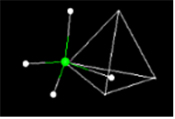## MathMol --Mathematics and Molecules

-------------->spin on -------->- spin off

spin on -- spin off-- stick-- ball-and-stick

To Rotate the Molecule--->Left Click and Drag
To Zoom In/Out-->>Left Click + hold Shift button and Drag Vertically (up/down)

MathMol (Mathematics and Molecules) is designed to serve as an introductory starting point for those interested in the field of molecular modeling. MathMol is an ideal STEM activity for K-12 students since it introduces molecular structure as it relates to mathematics and utilizes 3D visualization technology. The site is interactive not just in the software used but we also insert online question to test understanding.

MathMol was originally developed at the NYU Visualization lab in 1996, and tested in several accelerated middle school classrooms. Since most of the software originally used is currently outdated, MathMol is now in the process of being updated to HTML5 standards using Jsmol.

See Review from Education World --Top 20 Review of 1999

#### Activity1: Measuring length and distance at the molecular level

When we measure length or distance at the molecular level we are talking of a very small measurement. This activity introduces you to the software Jsmol and is used to measure line segments at the size of molecules.

#### Activity2: Geometry-of-1-Dimension

In this lesson we begin to take a look at the 3-Dimensions of the world we live in. In this activity we look at the geometry of 1-Dimension.

#### Activity3: Geometry of 2- Dimensions

When we see a graph on a page we really don't think in terms of dimensions. But the graph above has only two axis or two dimensions. It is a plane. ... see Geometry of 2-Dimensions

#### Activity4: Geometry of 3-Dimensions

Molecules are solid 3 dimensional objects in 3 dimensional space. To describe molecules accurately we must describe their postion in 3-D space.

#### Activity5: Introduction to Molecular Modeling using Jsmol

Using Jsmol you will view simple molecules -- water, methane, aspirin. Hydrocarbons Methane to Octane....

#### Activity7: Summary Sheet by Students

This is a summary sheet to test the students knowledge of previous activities...

#### Activity8: What is the Geometry of the Methane Molecule

We look at the geometry of the methane molecule in this interactive activity.

#### Activity9: Geometry of the Crystal Structure of Ice

Why does ice float... we look at the unique crystal structure of ice crystals.

#### Activity10: Geometry of the Benzene Molecule

The benzene molecule has a common geometric shape as does cyclohexane. You will measure angles and learn about the bonds in the benzene molecule.

#### MATHMOLK-12 STEM INTERACTIVE ACTIVITIES